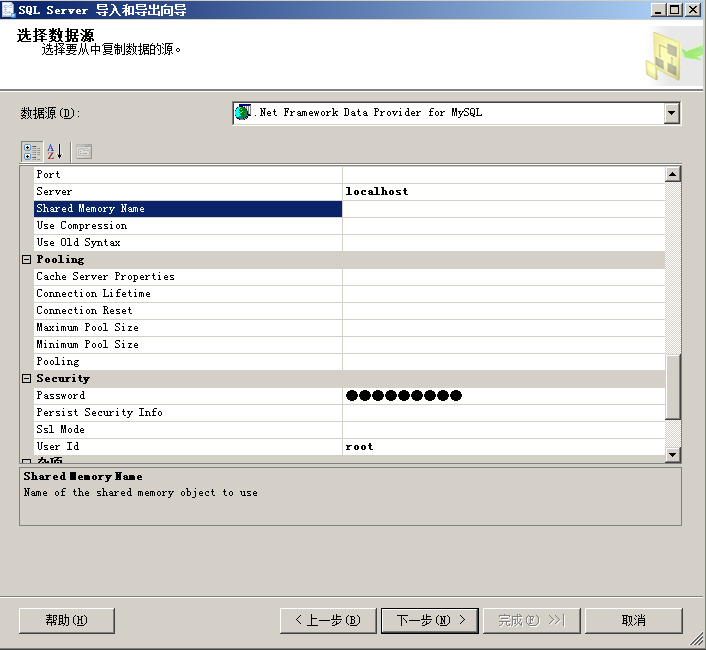MySQL(csv,text)导入mssql方法参考

csv/text导入MySQL

```#参考下面语句
\$ awk -F "\t" 'NF==63 {print \$0}' all.csv >all_63f.txt
\$ dos2unix h.txt       #注意最好不要带BOM，否则自行另存一下，避免麻烦

#最大计算字符长度，本身并不复杂，只是写在一行里，阅读不太方便
\$ awk -F "\t" 'BEGIN{for(i=1;i<=63;i++){xcount[i]=0}} {for(i=1;i<=63;i++){if(xcount[i]<length(\$i) ){xcount[i]=length(\$i)}}} END{for(i=1;i<=63;i++){print i,xcount[i]}}' all_63f.txt```

MySQL导入mssql

create table，建表，与mysql结构一致。coded by nessus